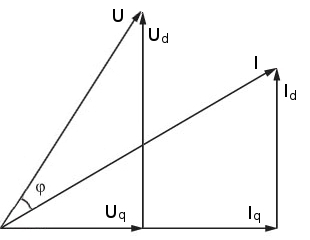# Power factor

Ratio between real power and apparent power.

The power factor cos(φ) is defined as the ratio between real power and apparent power consumed/produced by the electrical machine. The power factor is a dimensionless number between -1 and 1. In Emetor, the power factor cos(φ) is calculated from the phase angle φ between the phase current and the phase voltage, refer to Fig. 1:

$$\cos\left(\varphi\right) = \cos\left(\tan^{-1}\left( \frac{U_d}{U_q} \right)-\tan^{-1}\left( \frac{I_d}{I_q} \right)\right)$$Fig. 1 Illustration of the power factor calculation.

When designing an electrical motor, it is generally of interest to aim at a high power factor. In such a way, it can be avoided that the supply has to provide excessive reactive power to the motor, and the current necessary to obtain rated power can be effectively limited.